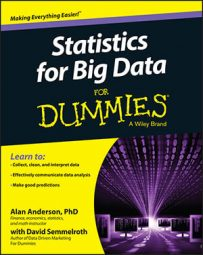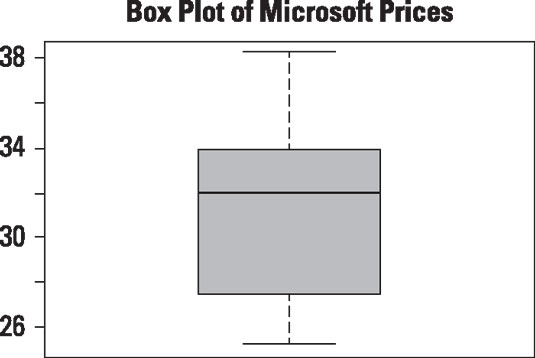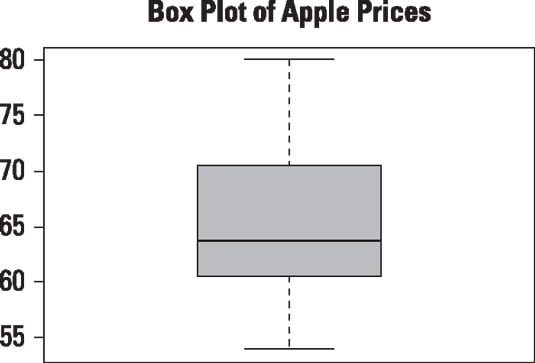##### Statistics for Big Data For DummiesA box plot is designed to show several key statistics for a dataset in the form of a vertical rectangle or box. The statistics it can show include the following:

• Minimum value

• Maximum value

• First quartile (Q1)

• Second quartile (Q2)

• Third quartile (Q3)

• Interquartile range (IQR)

The first quartile of a dataset is a numerical measure that divides the data into two parts: the smallest 25 percent of the observations and the largest 75 percent of the observations. In other words, the first quartile is a numerical value with the following properties:

• 25 percent of the observations in the dataset are smaller than the first quartile.

• 75 percent of the observations in the dataset are greater than the first quartile.

Similarly, the second quartile (also known as the median) divides the data in half, so 50 percent of the elements are smaller than the median, and 50 percent are larger.

The third quartile is the value for which the following are true:

• 75 percent of the observations in the dataset are smaller than the third quartile.

• 25 percent of the observations in the dataset are greater than the third quartile.

The interquartile range (IQR) is the difference between the third quartile and first quartile: IQR = Q3 – Q1.

The interquartile range is a measure of dispersion; it shows how much spread there is between the elements in the middle 50 percent of a dataset.

A box plot is drawn so that

• The top of the box represents the third quartile (Q3) of the data.

• The bottom of the box represents the first quartile (Q1) of the data.

• The middle of the box (shown with a line) represents the second quartile (Q2).

In addition, there's a line above the box to indicate the maximum value in the data that doesn't exceed Q3 + 1.5 x IQR and a line below the box to indicate the minimum value in the data that doesn't fall below Q1 – 1.5 x IQR. Values outside of this range are outliers and are shown on the box plot as individual points.

This figure shows a box plot of the daily prices of Microsoft stock from January 1, 2013 to December 31, 2013.Box plot of daily prices for Microsoft stock.

There are no outliers in this data. Therefore, the bottom line in the box plot shows that the lowest price during this period was somewhat less than \$26.00, and the top line shows that the highest price was just over \$38. The bottom of the box corresponds to the first quartile, which is \$27.43; the solid line in the middle of the box corresponds to the second quartile (median), which is \$31.89. The top of the box corresponds to the third quartile, which is \$33.78. The height of the box equals the interquartile range (IQR), which is \$6.35.

As another example, this figure shows a box plot of the daily prices of Apple stock from January 1, 2013 to December 31, 2013.Box plot of daily prices for Apple stock from January 1, 2013 to December 31, 2013.

The lowest price in 2013 for Apple stock was \$53.84, and the highest price was \$80.11. There are no outliers in the data, so these values are shown by the bottom line and top line, respectively.

The first quartile, shown at the bottom of the box, was \$60.48. The second quartile was \$63.65 (shown by the solid black line) and the third quartile was \$70.32, shown at the top of the box. As a result, the interquartile range (IQR) is \$9.84.